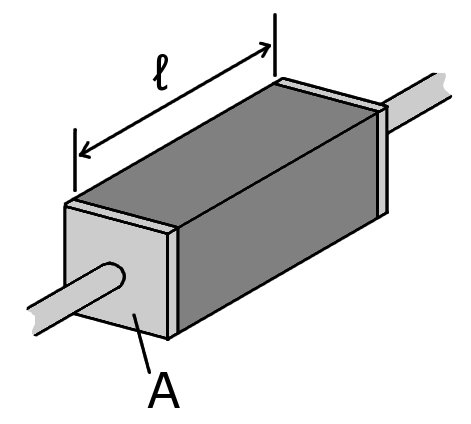# Difference Between Conductor and Insulator

## Main Difference – Conductor vs. Insulator

Conductor and Insulator are terms that describe whether a given material has properties favourable for conducting electricity or heat. The main difference between conductor and insulator is that a conductor conducts electricity or heat well, whereas an insulator conducts electricity or heat poorly. Based on whether we are interested in a material’s ability to conduct electricity or heat, we use terms electrical conductor/insulator or thermal conductor/insulator.

## What is a Conductor

thermal conductor conducts heat well. The rate of heat transfer,$\frac{\mathrm dQ}{\mathrm dt}$ or the heat current, between two objects having a temperature difference of$\Delta T$ is given by$\frac{\mathrm dQ}{\mathrm dt}=-\kappa\frac{A\Delta T}{l}$

where,$A$ and$l$ are the cross-sectional area and the length of the conductor transferring heat respectively. The letter$\kappa$ is called the thermal conductivity, measured in units of W m-1 K-1. This letter characterises the ability of the material to conduct heat. For instance, copper has a thermal conductivity of about 390 W m-1 K-1 while dry wood has a thermal conductivity of about 0.05 W m-1 K-1.

A material’s ability to conduct electricity is characterised by its electrical conductivity ($\sigma$), which is defined as the reciprocal of the material’s resistivity. That is,$\sigma=\frac{J}{E}$

where,$J$ is the current density and$E$ is the electric field strength. In actuality, the conductivity of a material is more often calculated using the formula$\sigma=\frac{l}{RA}$

where,$l$ is the length of the conductor and$A$ is the conductor’s cross-sectional area.$R$ is the conductor’s resistance, given by the ratio of the potential difference across the conductor to the current through the conductor. The units for measuring electrical conductivity is S m-1 (Siemens per metre). Copper has an electrical conductivity of about 5.9×107 S m-1. while lead has an electrical conductivity of about 4.6×106 S m-1.Dimensions used to calculate conductivity

In metals, electrons are mainly responsible for carrying current as well heat. Therefore, electrical and thermal conductivities are closely related. The relationship is given by the Wiedemann-Franz law:$\frac{\kappa}{\sigma}=LT$

where, T is the absolute temperature (in Kelvin) and$L$ is a constant called the Lorenz constant ($L=2.4\times 10^{-8}\mathrm{\:W\:\Omega\:K^{-2}}$).

The relationship between thermal and electrical conductivity for non-metals are not so clearly related: this is because electricity is always carried by free charge carriers whereas heat can be also conducted by vibrations of ions which are not free to move about. Typically, materials with metallic bonds are good thermal and electrical conductors, because they contain free electrons which can easily move about and conduct both electricity and heat.

## What is an Insulator

A material with a low thermal conductivity is called a thermal insulator. Glass is also a good insulator, with a thermal conductivity of about 0.8 W m-1 K-1. Air is an even better thermal insulator,  with a thermal conductivity of about 0.02 W m-1 K-1. Double-glazed glass makes use of the low thermal conductivity of air to insulate homes by having a layer of air trapped between two layers of glass.

Similarly, electrical insulators are materials with low electrical conductivities. PVC, which is used to insulate cables, has a very low conductivity of the order of 10-12 –  10-13 S m-1. Typically, materials made of polymers (having covalent bonds between them with very little free electrons) are good thermal and electrical insulators because most of their electrons are tightly bound.

## Difference Between Conductor and Insulator

Conductors are good at conducting heat and/or electricity

Insulators are not good at conducting heat and/or electricity.

The best conductors have many free carriers, such as electrons.

The best insulators do not have many free carriers.

Image Courtesy

“A somewhat cartoonish diagram of the geometry of the resistivity equation.” by User:Omegatron (Own work) [CC BY-SA 3.0], via Wikimedia Commons